# 力扣编译执行逻辑的大胆假设

## 起因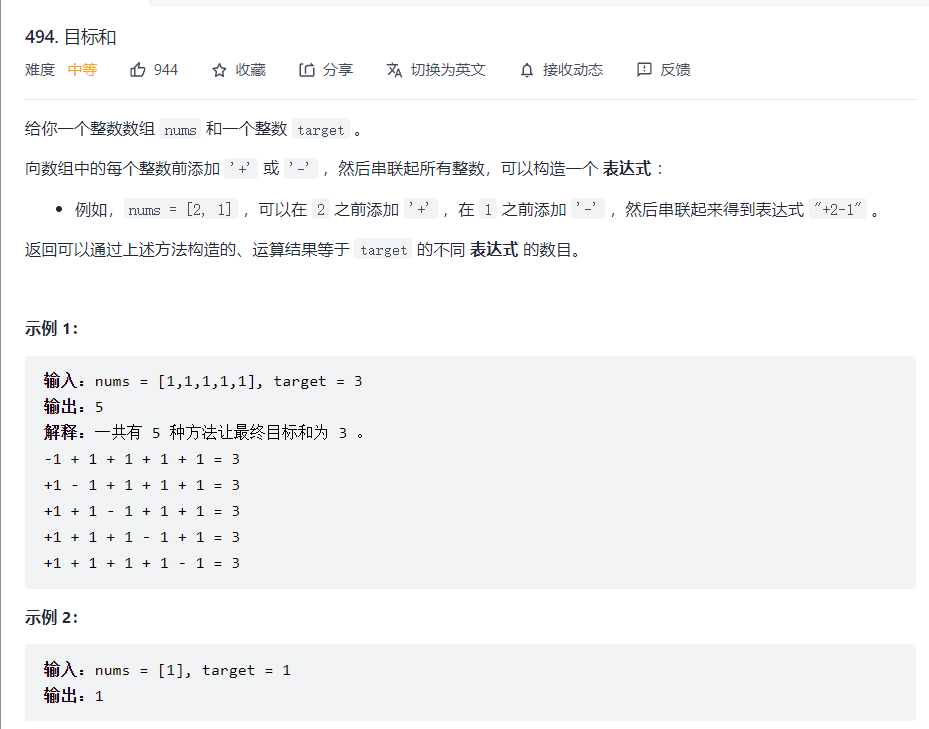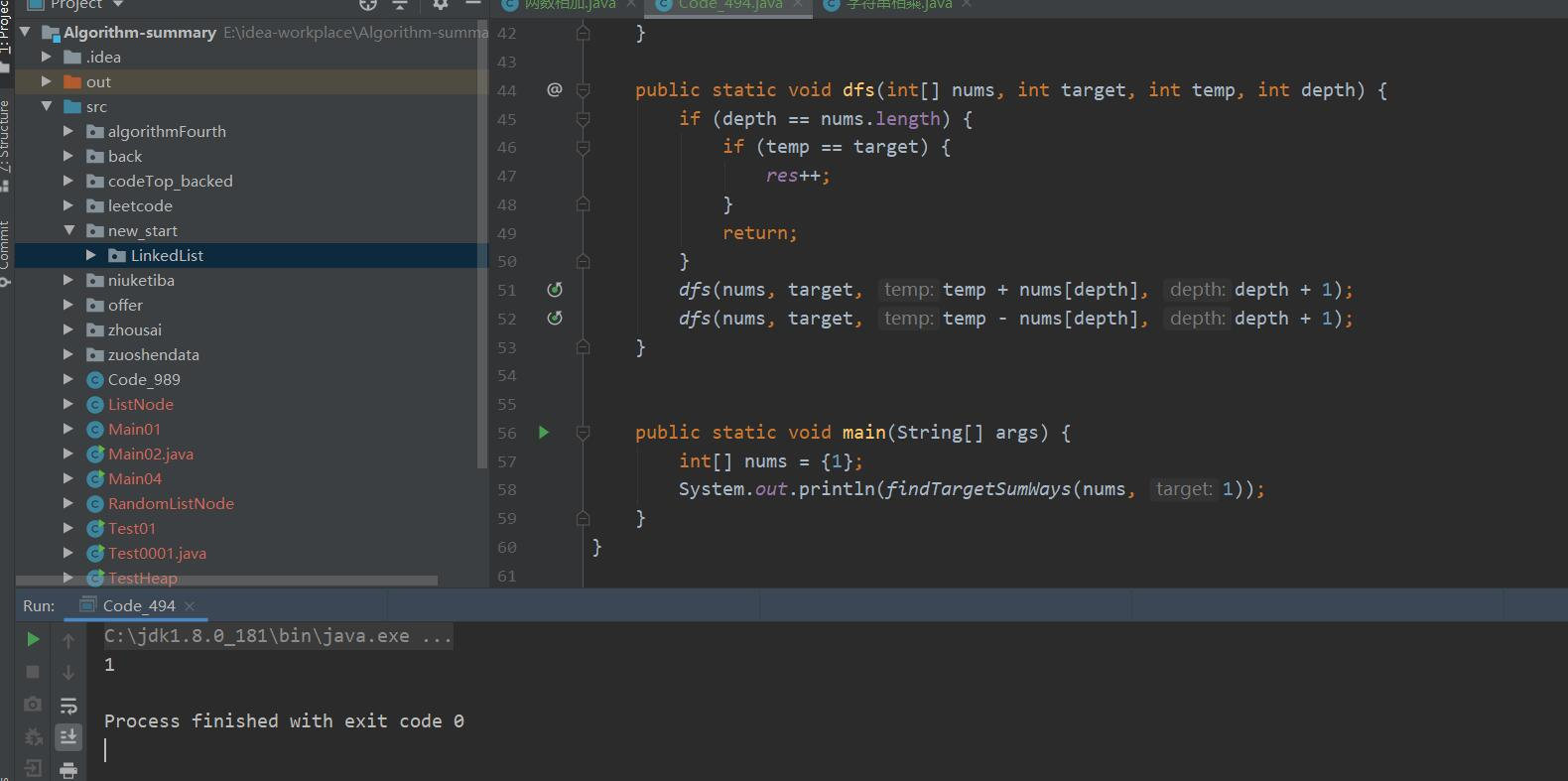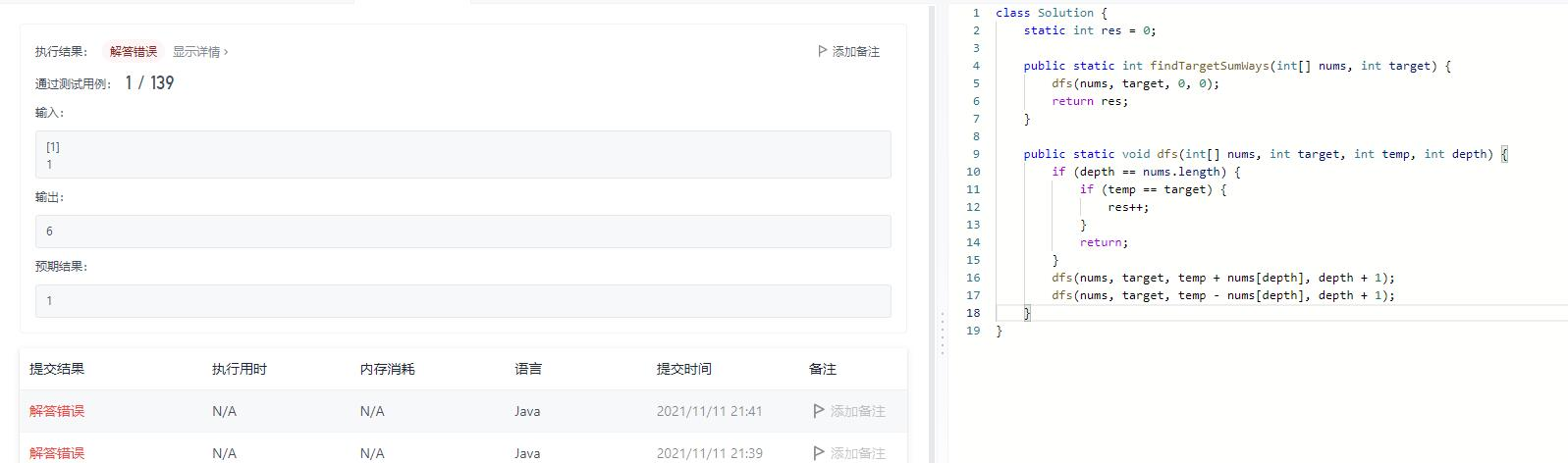## 解决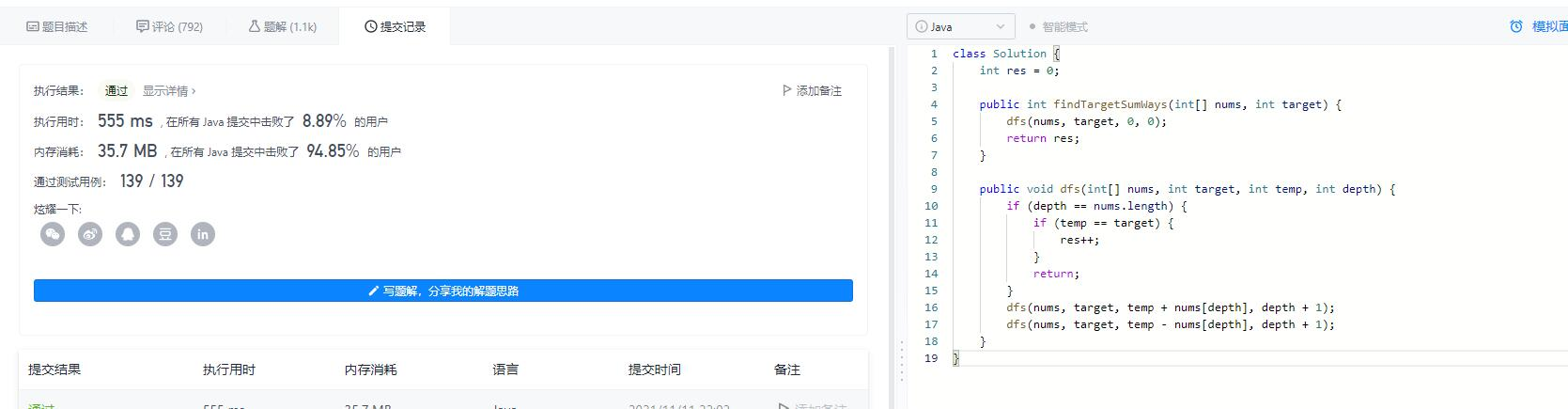## 大胆假设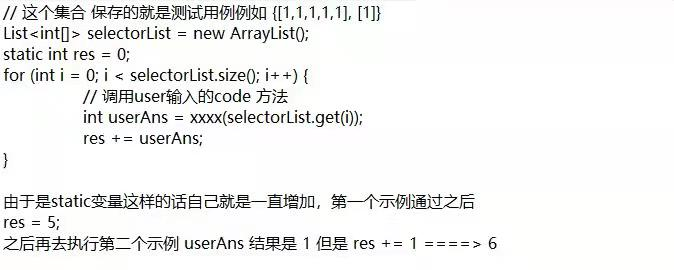## 验证

### 1.先不进行提交，直接进行测试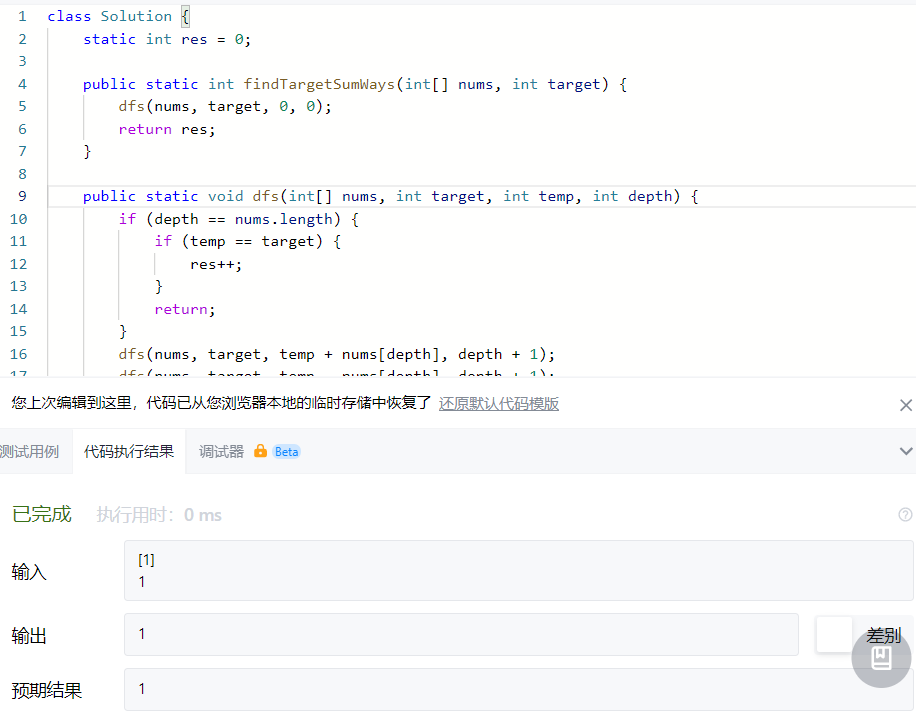==结果正确==

### 2. 进行提交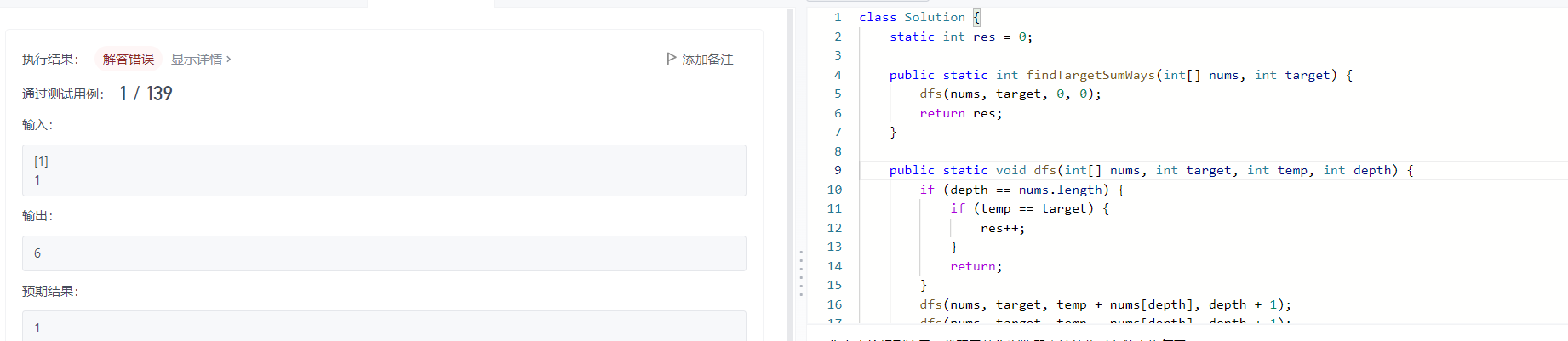==结果错误==

### 3. 跳过该用例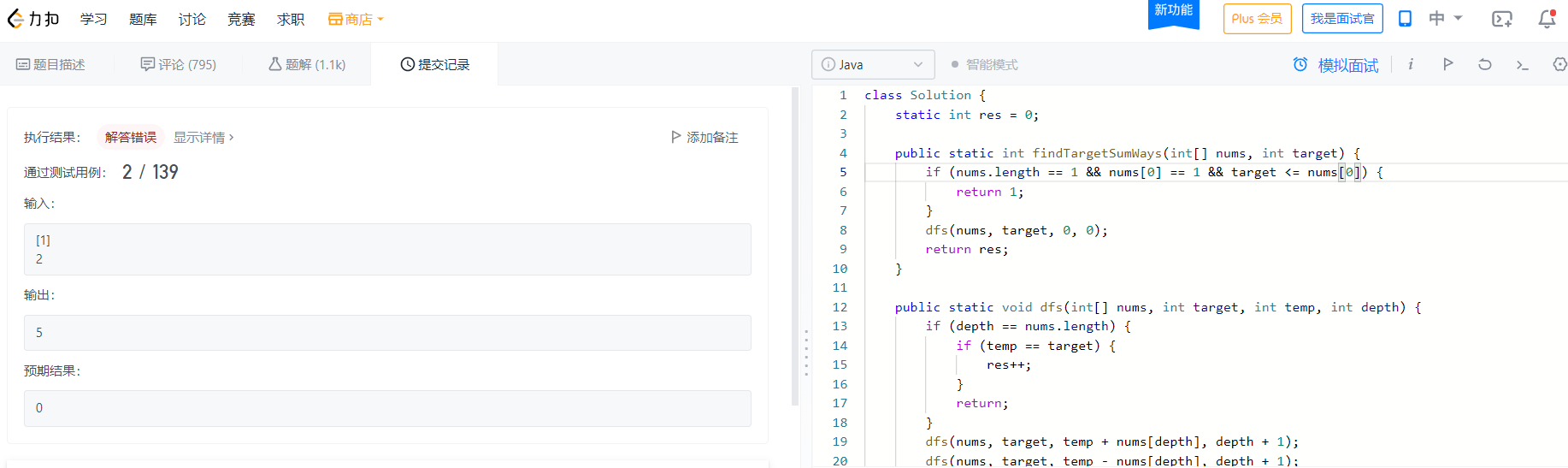res = 5 + 1 + 0 = 6

==结果错误==

### 4. 继续验证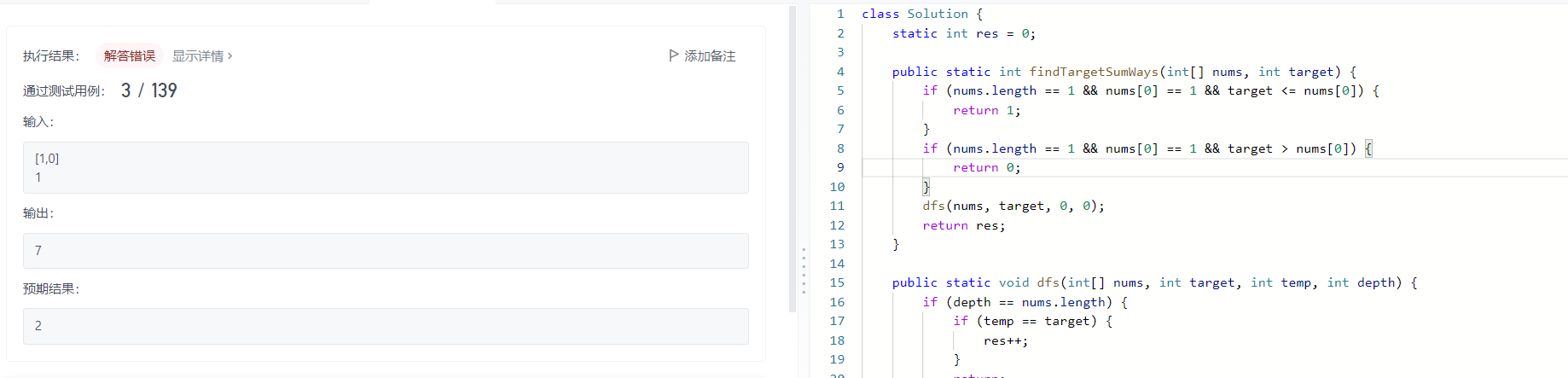res = 5 + 1 + 0 + 2 = 8
==结果错误==

## 代码验证

``````    static int res = 0;

public static int findTargetSumWays(int[] nums, int target) {
dfs(nums, target, 0, 0);
return res;
}

public static void dfs(int[] nums, int target, int temp, int depth) {
if (depth == nums.length) {
if (temp == target) {
res++;
}
return;
}
dfs(nums, target, temp + nums[depth], depth + 1);
dfs(nums, target, temp - nums[depth], depth + 1);
}

public static void main(String[] args) {
Map<int[], Integer> paramMap = new LinkedHashMap<>();
paramMap.put(new int[]{1, 1, 1, 1, 1}, 3);
paramMap.put(new int[]{1}, 1);
paramMap.put(new int[]{1}, 2);
paramMap.put(new int[]{1, 0}, 1);
paramMap.forEach((k, v) -> {
int num = findTargetSumWays(k, v);
for (int n : k) {
System.out.print(n + "\t");
}
System.out.println("target: " + v);
System.out.println("num: " + num);
System.out.println("res: " + res);
System.out.println("---------------------------------");
});
}
``````

### 运行结果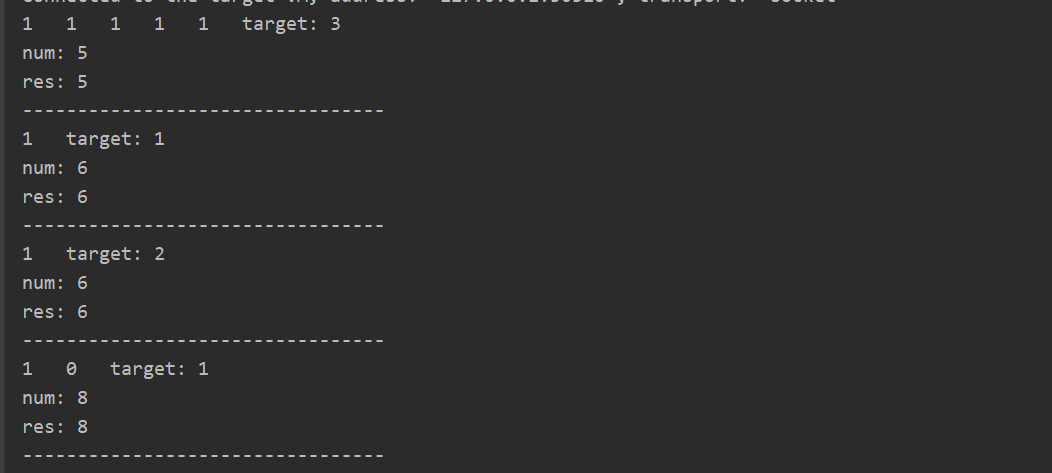1 Like